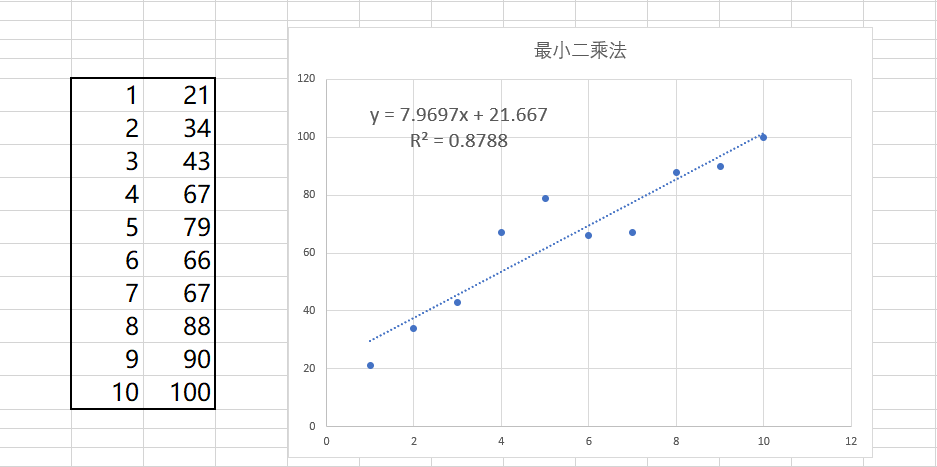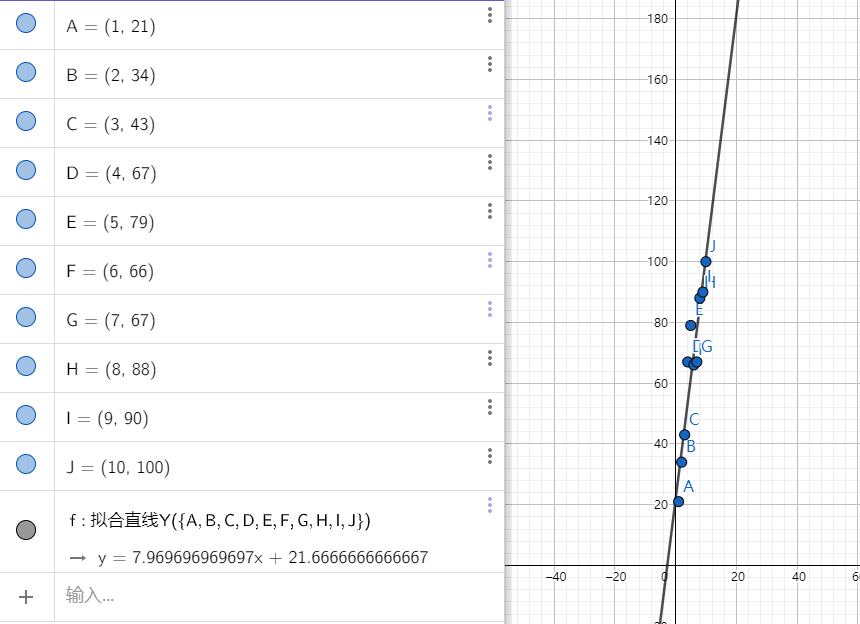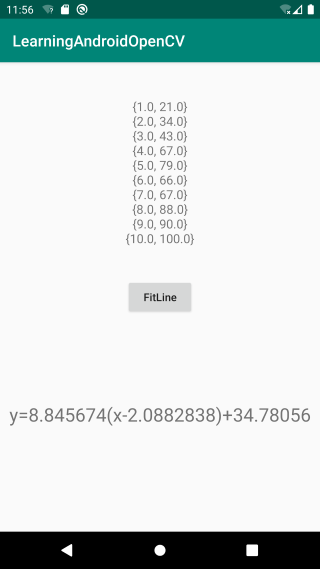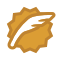# Android OpenCV（三十四）：直线拟合

## 概念

### 最小二乘法

$标函数=\sum（观测值-理论值）^2$

$(x_1,y_1),(x_2,y_2)……(x_n,y_n)$

$y=ax+b$

$e^2=\sum^n_{i=1}(y_i-y)^2\\ e^2=\sum^n_{i=1}(y_i-(ax_i+b))^2\\$

$\frac{\partial e}{\partial a} = \sum^n_{i=1}2(y_i-(ax_i+b)(-x_i))=0\\ = \sum^n_{i=1}(ax_i^2+bx_i-y_ix_i)=0\\$

$得到等式\\（\sum^n_{i=1}x^2_i）a+(\sum^n_{i=1})b=\sum^n_{i=1}y_ix_i\\ \frac{\partial e}{\partial b} = \sum^n_{i=1}2(y_i-(ax_i+b)(-1))=0\\ =\sum^n_{i=1}(ax_i+b-y_i)=0\\$

得到等式\\（\sum^n_{i=1}x_i）a+nb=\sum^n_{i=1}y_i\\ \left\{ \begin{aligned} a\sum^n_{i=1}x_i&=\sum^n_{i=1}y_i-bn &(1)\\ a\sum^n_{i=1}x_i^2&=\sum^n_{i=1}x_iy_i-b\sum^n_{i=1}x &(2)\\ \end{aligned} \right.\\

$(1)/(2)得到\\ \frac{\sum^n_{i=1}x_i}{\sum^n_{i=1}x_i^2}=\frac{\sum^n_{i=1}y_i-bn}{\sum^n_{i=1}x_iy_i-b\sum^n_{i=1}x} (3)\\$

$(3)交叉相乘后化简\\ \sum^n_{i=1}x_i\sum^n_{i=1}x_iy_i-b(\sum^n_{i=1}x_i)^2=\sum^n_{i=1}x_i^2\sum^n_{i=1}y_i-bn\sum^n_{i=1}x_i^2(4)\\$

通过(4)求得\\ \left\{ \begin{aligned} a&=\frac{\sum^n_{i=1}x_i\sum^n_{i=1}y_i-n\sum^n_{i=1}x_iy_i}{(\sum^n_{i=1}x_i)^2-n\sum^n_{i=1}x_i^2}\\ b&=\frac{\sum^n_{i=1}x_i\sum^n_{i=1}x_iy_i-\sum^n_{i=1}x_i^2\sum^n_{i=1}y_i}{(\sum^n_{i=1}x_i)^2-n\sum^n_{i=1}x_i^2}\\ \end{aligned} \right.\\

### Excel直线拟合### GeoGebra 直线拟合## API

public static void fitLine(Mat points, Mat line, int distType, double param, double reps, double aeps)

• 参数一：points，待拟合直线的点集。2D或3D点的输入向量，存储在std :: vector <>或Mat中

• 参数二：line，输出线路参数。如果是2D拟合，则它应该是4个元素的向量（例如Vec4f）-（vx，vy，x0，y0），其中（vx，vy）是与线共线的归一化向量，而（x0，y0 ）是直线上的一点。如果是3D拟合，则它应该是6个元素的向量（例如Vec6f）-（vx，vy，vz，x0，y0，z0），其中（vx，vy，vz）是与线共线的归一化向量和（x0，y0，z0）是直线上的一点

• 参数三：distType，最小二乘法使用的距离类型标志。

enum DistanceTypes {
DIST_USER    = -1,  //!< User defined distance
DIST_L1      = 1,   //!< distance = |x1-x2| + |y1-y2|
DIST_L2      = 2,   //!< the simple euclidean distance
DIST_C       = 3,   //!< distance = max(|x1-x2|,|y1-y2|)
DIST_L12     = 4,   //!< L1-L2 metric: distance = 2(sqrt(1+x*x/2) - 1))
DIST_FAIR    = 5,   //!< distance = c^2(|x|/c-log(1+|x|/c)), c = 1.3998
DIST_WELSCH  = 6,   //!< distance = c^2/2(1-exp(-(x/c)^2)), c = 2.9846
DIST_HUBER   = 7    //!< distance = |x|<c ? x^2/2 : c(|x|-c/2), c=1.345
};

• 参数四：param，某些距离类型的数值参数（C）。如果为0，则会自动选择一个最佳值

• 参数五：reps，坐标原点与拟合直线之间的距离精度，数值为0表示选择自适应参数，一般选择0.01

• 参数六：aeps，拟合直线的角度精度，数值0表示选择自适应参数，一般选择0.01

### 标志位计算公式

• DIST_L1
$\rho (r) = r$

• DIST_L2
$\rho (r) = r^2/2 \quad \text{(the simplest and the fastest least-squares method)}$

• DIST_L12
$\rho (r) = 2 \cdot ( \sqrt{1 + \frac{r^2}{2}} - 1)$

• DIST_FAIR
$\rho \left (r \right ) = C^2 \cdot \left ( \frac{r}{C} - \log{\left(1 + \frac{r}{C}\right)} \right ) \quad \text{where} \quad C=1.3998$

• DIST_WELSCH
$\rho \left (r \right ) = \frac{C^2}{2} \cdot \left ( 1 - \exp{\left(-\left(\frac{r}{C}\right)^2\right)} \right ) \quad \text{where} \quad C=2.9846$

• DIST_HUBER
\rho \left (r \right )= \left\{ \begin{aligned} &\frac{r^2}{2},&当r

## 操作

/**
* 直线拟合
* author: yidong
* 2020/9/8
*/
class FitLineActivity : AppCompatActivity() {

private lateinit var mBinding: ActivityFitLineBinding
private val mPoints = MatOfPoint(
Point(1.0, 21.0),
Point(2.0, 34.0),
Point(3.0, 43.0),
Point(4.0, 67.0),
Point(5.0, 79.0),
Point(6.0, 66.0),
Point(7.0, 67.0),
Point(8.0, 88.0),
Point(9.0, 90.0),
Point(10.0, 100.0)
)

override fun onCreate(savedInstanceState: Bundle?) {
super.onCreate(savedInstanceState)
mBinding = DataBindingUtil.setContentView(this, R.layout.activity_fit_line)
mBinding.presenter = this

val points = StringBuilder()
for (point in mPoints.toList()) {
points.append(point.toString() + "\n")
}
mBinding.tvPoints.text = points
}

fun doFitLine() {
val result = Mat()
Imgproc.fitLine(mPoints, result, Imgproc.DIST_L1, 0.0, 0.00, 0.00)
val lines = FloatArray(4)
val tmp = FloatArray(1)
for (i in 0 until result.rows()) {
result.get(i, 0, tmp)
lines[i] = tmp
}
val k = lines / lines
mBinding.tvResult.text = "y=$k(x-${lines})+\${lines}"
result.release()
}
}


## 效果## 源码

https://github.com/onlyloveyd/LearningAndroidOpenCV

## 扫码关注，持续更新

• 【计算机视觉】
• 【Android】
• 【Flutter】
• 【数字图像处理】
• 【计算机视觉算法与应用】onlyloveydCSDN认证博客专家 Android Kotlin OpenCV

08-071万+

#### Android OpenCV 实例笔记3 -- 摄像头竖屏全屏的设置,更新完整代码

04-187481

#### opencv 直线拟合03-211018

#### Android OpenCV Camera preview 横屏以及不全屏的问题

06-23173

#### Android 调整屏幕分辩率

03-302245

#### 【OpenCV】opencv 检测直线、圆、矩形

10-162397

#### OpenCV for Android 设置预览界面尺寸

04-073万+

#### 【OpenCV3】直线拟合——cv::fitLine()详解

07-26793

#### Android Studio4.0+OpenCv4.3.0实战之SVM算法

04-141666

#### OpenCV的作用及安装

06-105549

#### Android平台上基于OpenCV的道路循迹©️2020 CSDN 皮肤主题: 数字20 设计师: CSDN官方博客点击重新获取扫码支付1.余额是钱包充值的虚拟货币，按照1:1的比例进行支付金额的抵扣。
2.余额无法直接购买下载，可以购买VIP、C币套餐、付费专栏及课程。余额充值